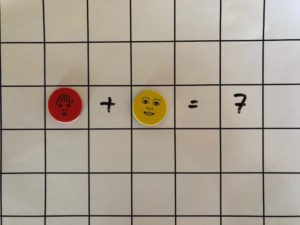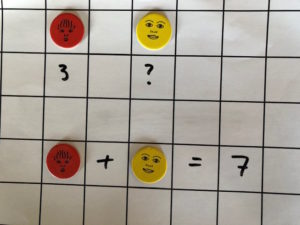# Encouraging challenging problem solving in elementary mathematics

posted on December 17, 2018

Through the use of classroom manipulatives, we can encourage a wide range of thinking and questioning at the elementary level. Using sets of diskii where each of ten tokens has a unique name, face, and color, we can avail students of opportunities to work collaboratively to solve hands-on problems. The opportunity exists to vary the complexity of the challenge according to the topic or grade being taught.

In a very simple example, consider the equation 3+4=7. We could ask students what 3+4 equals. There is one finite answer. However, using diskii we can ask what the possible values of the tokens are if: token + token = 7. Already, the complexity of the challenge is increased. Alternatively, we could set the value of one token at 3, and ask what the value of the remaining token is if: token + token = 7 given that one token has a value of 3. Here, students have to retrieve information and apply that knowledge (two critical learning skills) to solving the problem.

diskii HERE